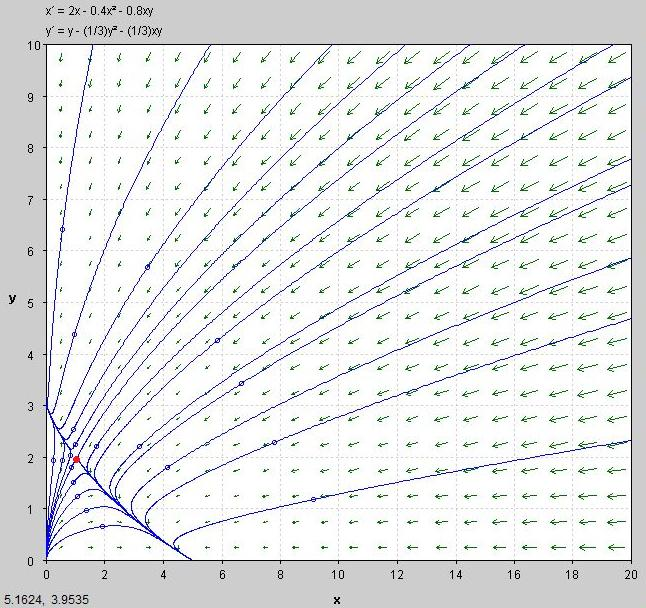#### You may also like### Ball Bearings

If a is the radius of the axle, b the radius of each ball-bearing, and c the radius of the hub, why does the number of ball bearings n determine the ratio c/a? Find a formula for c/a in terms of n.### Overarch 2

Bricks are 20cm long and 10cm high. How high could an arch be built without mortar on a flat horizontal surface, to overhang by 1 metre? How big an overhang is it possible to make like this?### Cushion Ball

The shortest path between any two points on a snooker table is the straight line between them but what if the ball must bounce off one wall, or 2 walls, or 3 walls?

# Population Dynamics - Part 6

##### Age 16 to 18 Challenge Level:

We now incorporate the effect of the environment on the Lotka-Volterra equations derived earlier. Consider a population of giraffes of size x and a population of hyenas of size y.

Using the logistic equation from before, we can model the effect of the carrying capacity with the equations:  \begin{align*} \frac {\mathrm{d}x}{\mathrm{d}t}&=r_1 \frac{K_1-x}{K_1} x= r_1 x\left(1-\frac{x}{K_1}\right) \\ \frac {\mathrm{d}y}{\mathrm{d}t}&=r_2 \frac{K_2-y}{K} = r_2y\left(1-\frac{y}{K_2}\right) \end{align*} An increase in either population of species will reduce the resources available to both. In order to model this, we introduce a competition coefficient to represent the competitive effect of one species on the other.

Let $\alpha$ be the competitive effect of the hyenas on the giraffes, and $\beta$ be the competitive effect of the giraffes on the hyenas. We then consider the terms  $\frac{K_1-x-\alpha y}{K_1}$ and $\frac{K_2-y-\beta x}{K_2}$ .

Question:   Can you explain the logic behind these terms? Think what would happen if either the giraffe or hyena population died out.

Our population equations then become: \begin{align*} \frac {\mathrm{d}x}{\mathrm{d}t}&=r_1 x \Bigg(1- \frac{x+\alpha y}{K_1}\Bigg) \\ \frac {\mathrm{d}y}{\mathrm{d}t}&=r_2 y \Bigg(1- \frac{y+\beta x}{K_2}\Bigg) \end{align*}

Question:    Suppose (quite grandly) that two populations of giraffes and hyenas have population equations  $\frac {\mathrm{d}x}{\mathrm{d}t}=2x\bigg(1-\frac{x+2y}{5}\bigg)$ and $\frac {\mathrm{d}y}{\mathrm{d}t}=y\bigg(1-\frac{y+x}{3}\bigg)$ .

What are the equilibrium points?

Look at the phase diagram below. What is happening to the populations of both animals? What do you think the red point means?Question:    If you were to create your own model, what other parameters would you consider when creating differential equations to describe the population sizes? Perhaps think about other predators and seasonal variation of the carrying capacity.